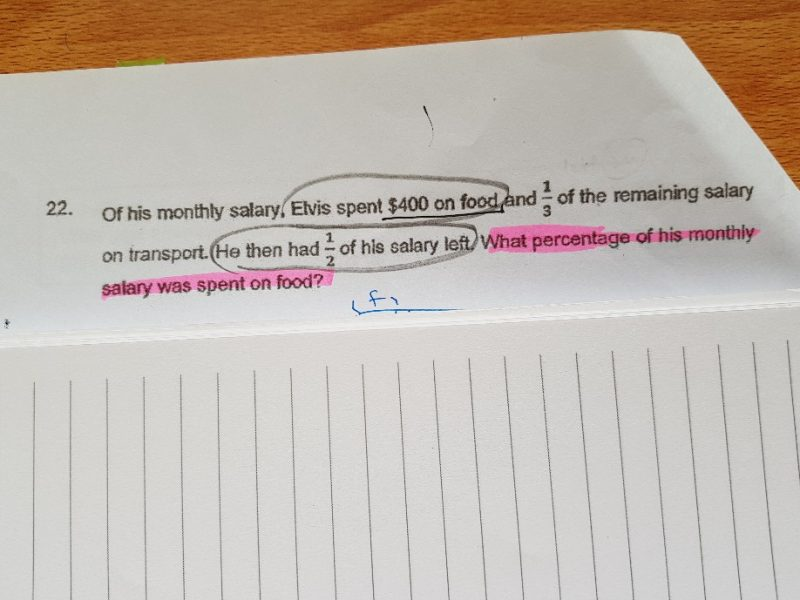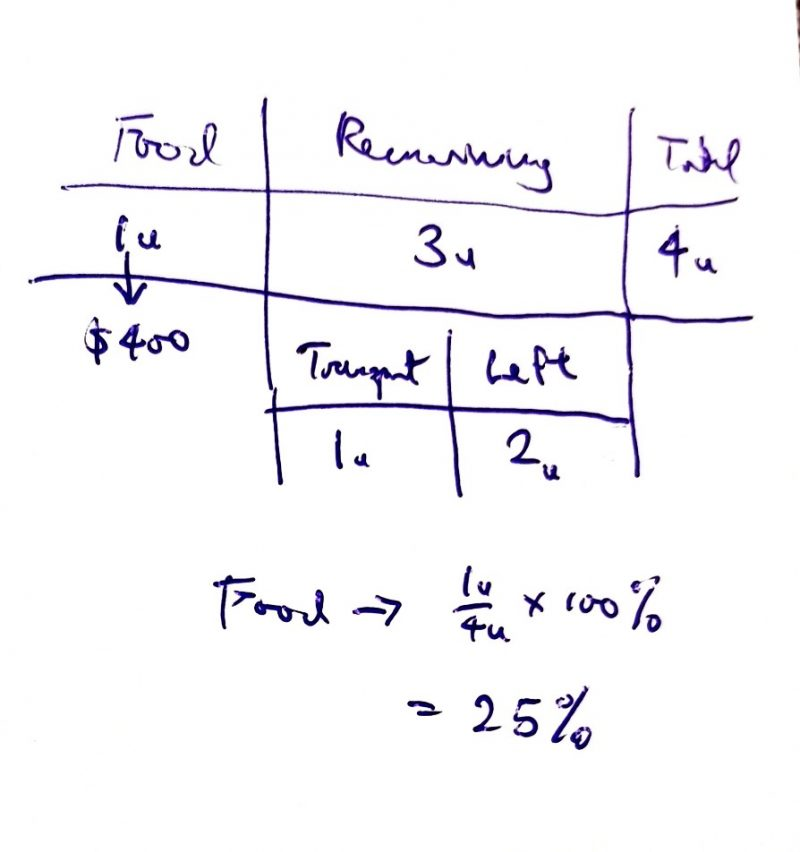# Question1 Reply 0 Likes

Hi thw,

How to you know \$400 is one unit?

1 Reply 0 Likes

Transport is 1/3 of the remaining amount so what is left must be 2u. Since what is left is 1/2 = 2/4 of the original amount, the total must be 4 units. Since the remaining part is 3 units, Food must be 4 units – 3 units = 1 unit (= \$400).

1 Reply 0 Likes

Hi thw,

Knew it.

Thank you.

0 Replies 0 Likes